# Plotting MCMC draws using the bayesplot package

#### 2017-04-12

This vignette focuses on plotting parameter estimates from MCMC draws. MCMC diagnostic plots are covered in the separate vignette Visual MCMC diagnostics using the bayesplot package, and graphical posterior predictive checks are covered in Graphical posterior predictive checks using the bayesplot package.

## Plots for MCMC draws

The bayesplot package provides various plotting functions for visualizing Markov chain Monte Carlo (MCMC) draws from the posterior distribution of the parameters of a Bayesian model.

In this vignette we’ll use draws obtained using the `stan_glm` function in the rstanarm package (Gabry and Goodrich, 2016), but MCMC draws from using any package can be used with the functions in the bayesplot package. See, for example, brms (which, like rstanarm, calls the rstan package internally to use Stan’s MCMC sampler).

``````library("rstanarm")
fit <- stan_glm(
mpg ~ ., # ~ . includes all other variables in dataset
data = mtcars,
chains = 4,
iter = 2000,
seed = 1111
)
print(fit)``````
``````stan_glm
family:  gaussian [identity]
formula: mpg ~ .
------

Estimates:
(Intercept) 12.2   18.2
cyl         -0.1    1.1
disp         0.0    0.0
hp           0.0    0.0
drat         0.8    1.7
wt          -3.6    2.0
qsec         0.8    0.7
vs           0.3    2.2
am           2.5    2.1
gear         0.7    1.6
carb        -0.3    0.9
sigma        2.8    0.4

Sample avg. posterior predictive
distribution of y (X = xbar):
mean_PPD 20.1    0.7

------
For info on the priors used see help('prior_summary.stanreg').``````

To use the posterior draws with the functions in the bayesplot package we’ll extract them from the fitted model object:

``````posterior <- as.array(fit)
dim(posterior)``````
`` 1000    4   12``
``dimnames(posterior)``
``````\$iterations
NULL

\$chains
 "chain:1" "chain:2" "chain:3" "chain:4"

\$parameters
 "(Intercept)" "cyl"         "disp"        "hp"          "drat"
 "wt"          "qsec"        "vs"          "am"          "gear"
 "carb"        "sigma"      ``````

### Interval estimates

Posterior intervals for the parameters can be plotted using the `mcmc_intervals` function.

``````library("bayesplot")
color_scheme_set("red")
mcmc_intervals(posterior, pars = c("cyl", "drat", "am", "sigma"))``````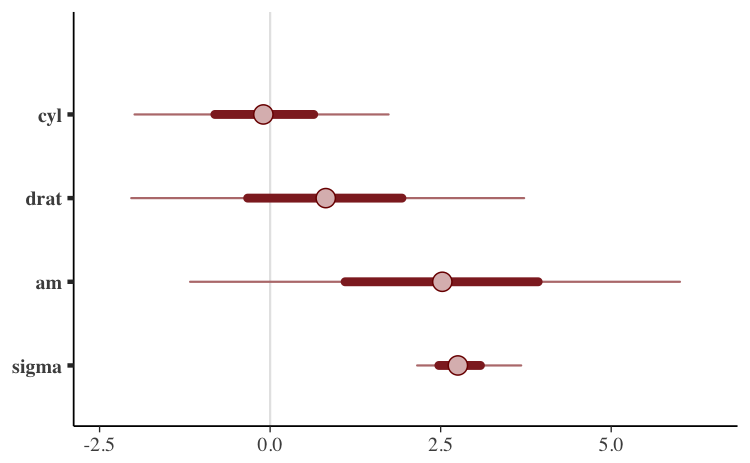The default is to show 50% intervals (the thick lines) and 90% intervals (the thinner outer lines). These defaults can be changed using the `prob` and `prob_outer` arguments, respectively. The points in the above plot are posterior medians. The `point_est` argument can be used to select posterior means instead or to omit the point estimates.

To show the uncertainty intervals as shaded areas under the estimated posterior density curves we can use the `mcmc_areas` function:

``````mcmc_areas(
posterior,
pars = c("cyl", "drat", "am", "sigma"),
prob = 0.8, # 80% intervals
prob_outer = 0.99, # 99%
point_est = "mean"
)``````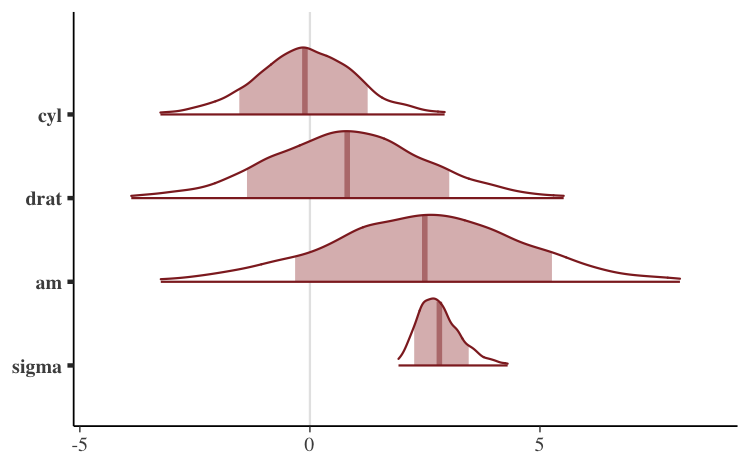### Histograms and density estimates

Histograms or kernel density estimates of posterior distributions of the various model parameters can be visualized using the functions described on the `MCMC-distributions` page in the bayesplot documentation.

#### Histograms

The `mcmc_hist` and `mcmc_dens` functions plot posterior distributions (combining all chains):

``````color_scheme_set("green")
mcmc_hist(posterior, pars = c("wt", "am"))``````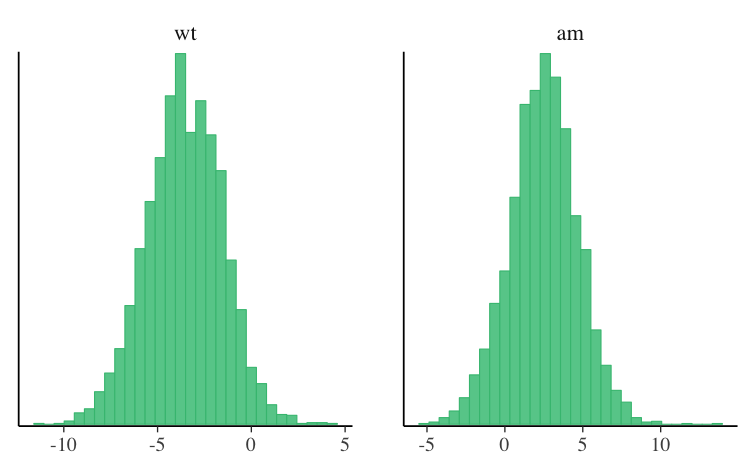``mcmc_dens(posterior, pars = c("wt", "am"))``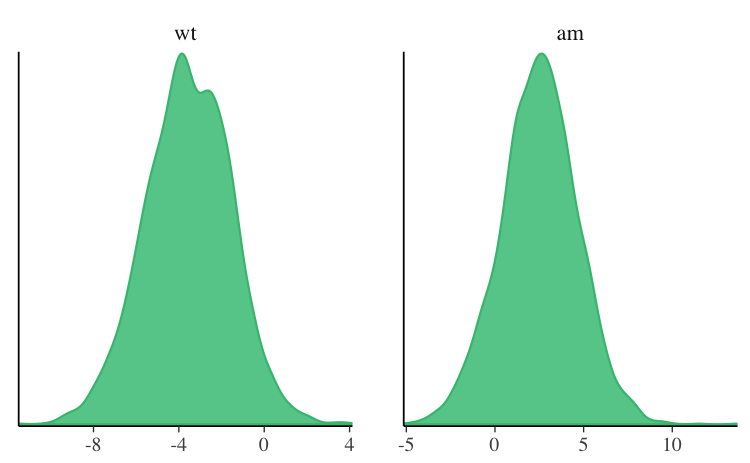To view the four Markov chain separately we can use `mcmc_hist_by_chain`, `mcmc_dens_overlay`:

``````color_scheme_set("brightblue")
mcmc_hist_by_chain(posterior, pars = c("wt", "am"))``````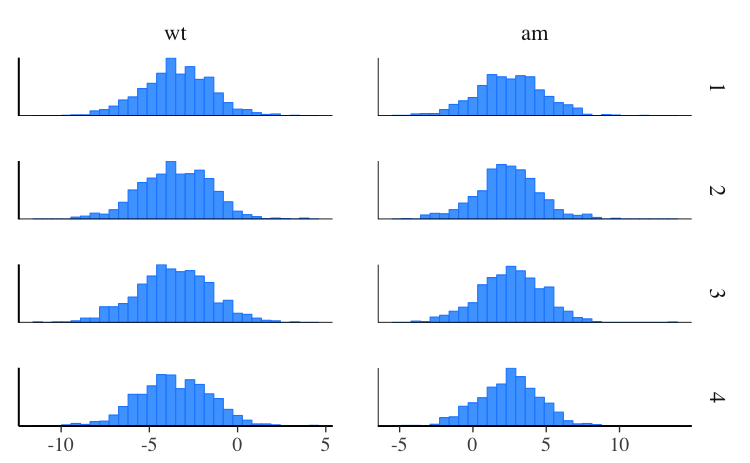``mcmc_dens_overlay(posterior, pars = c("wt", "am"))``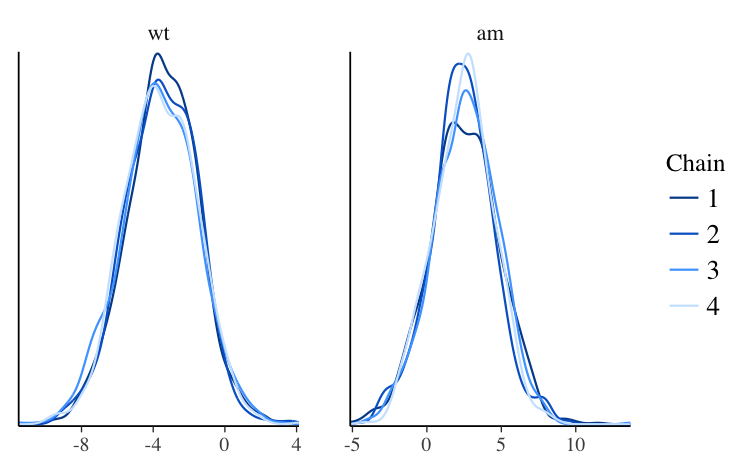The `mcmc_violin` function also plots the density estimates of each chain as violins with horizontal lines at user-specified quantiles:

``mcmc_violin(posterior, pars = c("wt", "am"), probs = c(0.1, 0.5, 0.9))``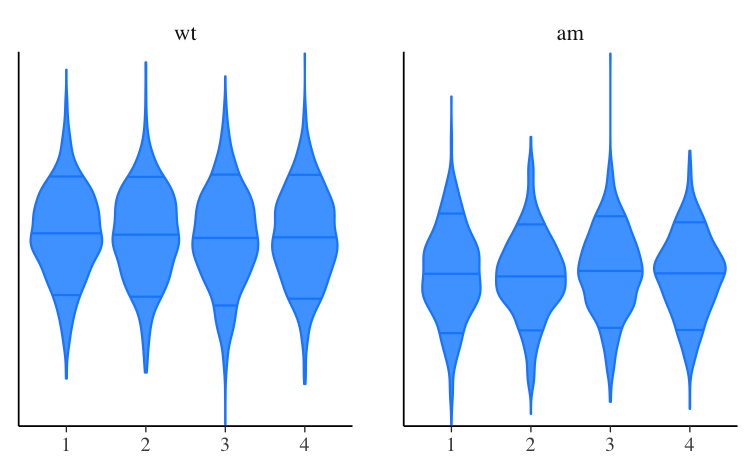### Scatterplots

The `mcmc_scatter` function creates a scatterplot with two parameters:

``````color_scheme_set("gray")
mcmc_scatter(posterior, pars = c("(Intercept)", "wt"), size = 1.5, alpha = 0.5)``````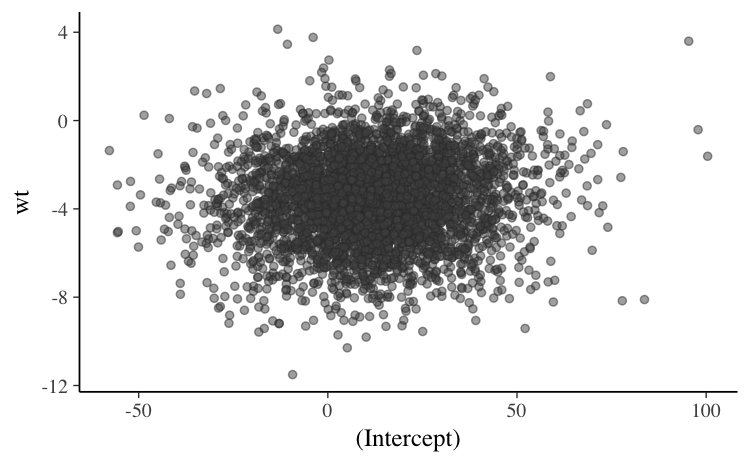The `mcmc_hex` function creates a similar plot but using hexagonal binning, which can be useful to avoid overplotting:

``mcmc_hex(posterior, pars = c("(Intercept)", "wt"))``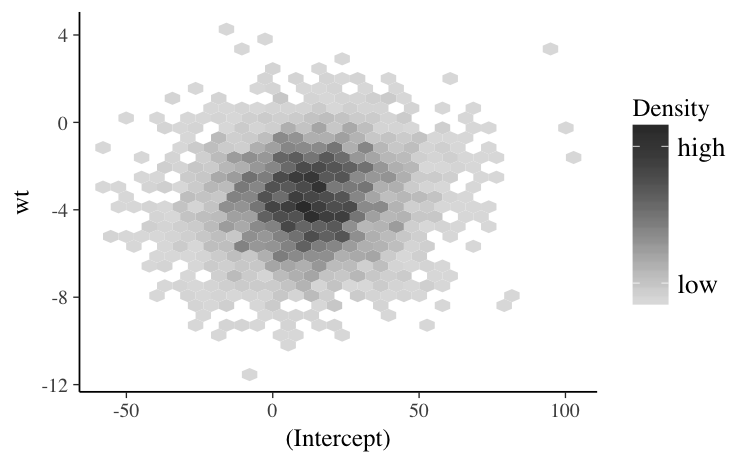In addition to `mcmc_scatter` and `mcmc_hex`, as of bayesplot version 1.2.0 an `mcmc_pairs` function for creating pairs plots with more than two parameters is available. Examples will eventually be included in this vignette. For now see `help("mcmc_pairs")`.

### Traceplots

The `mcmc_trace` function creates standard traceplots:

``````color_scheme_set("blue")
mcmc_trace(posterior, pars = c("wt", "sigma"))``````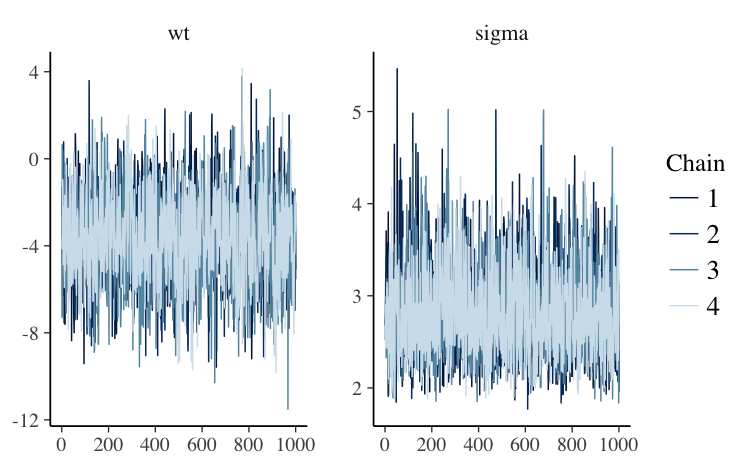If it’s hard to see the difference between the chains we can change to a mixed color scheme, for example:

``````color_scheme_set("mix-blue-red")
mcmc_trace(posterior, pars = c("wt", "sigma"),
facet_args = list(ncol = 1, strip.position = "left"))``````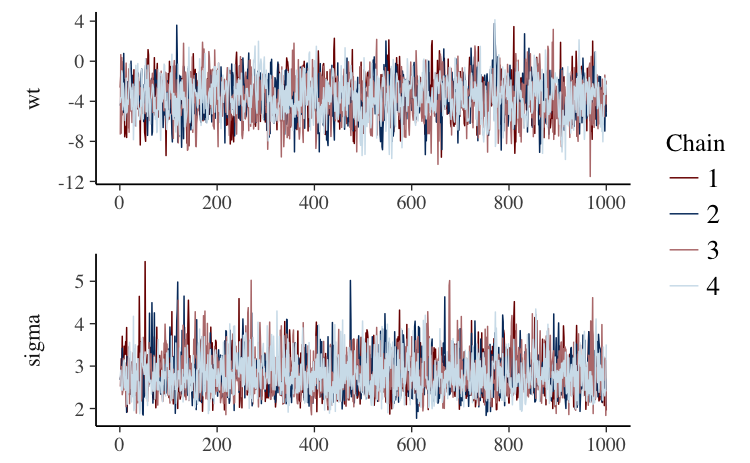The code above also illustrates the use of the `facet_args` argument, which is a list of parameters passed to `facet_wrap` in ggplot2. Specifying `ncol=1` means the traceplots will be stacked in a single column rather than placed side by side, and `strip.position="left"` moves the facet labels to the y-axis (instead of above each facet).

The `"viridis"` color scheme is also useful for traceplots because it is comprised of very distinct colors:

``````color_scheme_set("viridis")
mcmc_trace(posterior, pars = "(Intercept)")``````